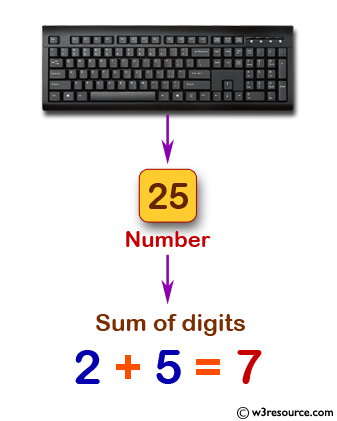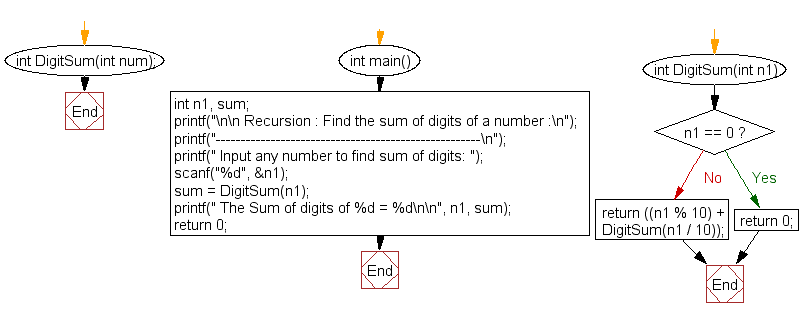﻿ C Program: Find the sum of digits of a number - w3resource

# C Exercises: Find the sum of digits of a number

## C Recursion: Exercise-6 with Solution

Write a program in C to find the sum of digits of a number using recursion.

Pictorial Presentation:Sample Solution:

C Code:

``````#include <stdio.h>

int DigitSum(int num);

int main()
{
int n1, sum;
printf("\n\n Recursion : Find the sum of digits of a number :\n");
printf("-----------------------------------------------------\n");
printf(" Input any number to find sum of digits: ");
scanf("%d", &n1);

sum = DigitSum(n1);//call the function for calculation

printf(" The Sum of digits of %d = %d\n\n", n1, sum);

return 0;
}

int DigitSum(int n1)
{
if(n1 == 0)
return 0;

return ((n1 % 10) + DigitSum(n1 / 10));//calling the function DigitSum itself
}
```
```

Sample Output:

``` Recursion : Find the sum of digits of a number :
-----------------------------------------------------
Input any number to find sum of digits: 25
The Sum of digits of 25 = 7
```

Explanation:

```int DigitSum(int n1)
{
if(n1 == 0)
return 0;

return ((n1 % 10) + DigitSum(n1 / 10));//calling the function DigitSum itself
}
```

The function ‘DigitSum()’ takes an integer ‘n1’ as input and calculates the sum of its digits recursively.

The function first checks if the input n1 is equal to 0. If it is, then the function returns 0 as the sum of digits for an empty number is 0.

If the input n1 is not equal to 0, then the function calculates the remainder of the number divided by 10 (which gives the last digit of the number) and adds it to the sum of the remaining digits obtained by calling DigitSum() recursively with the number divided by 10 (which removes the last digit).

The function keeps calling itself recursively until the number becomes 0, at which point the sum of all the digits is returned.

Time complexity and space complexity:

The time complexity of the function is O(log n) where n is the given number.

The space complexity of the function is O(log n) due to the recursive calls.

Flowchart:C Programming Code Editor:

Have another way to solve this solution? Contribute your code (and comments) through Disqus.

What is the difficulty level of this exercise?

Test your Programming skills with w3resource's quiz.

﻿

## C Programming: Tips of the Day

__FILE__ macro shows full path

```#include <string.h>

#define __FILENAME__ (strrchr(__FILE__, '/') ? strrchr(__FILE__, '/') + 1 : __FILE__)
For Windows use '\\' instead of '/'.
```

Ref : https://bit.ly/3iEWRoT

We are closing our Disqus commenting system for some maintenanace issues. You may write to us at reach[at]yahoo[dot]com or visit us at Facebook